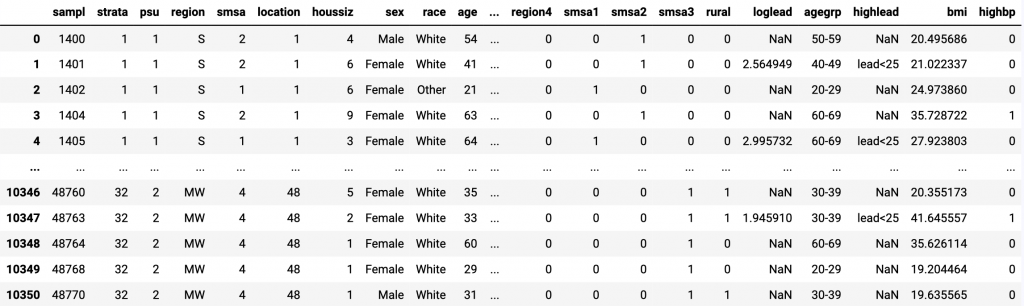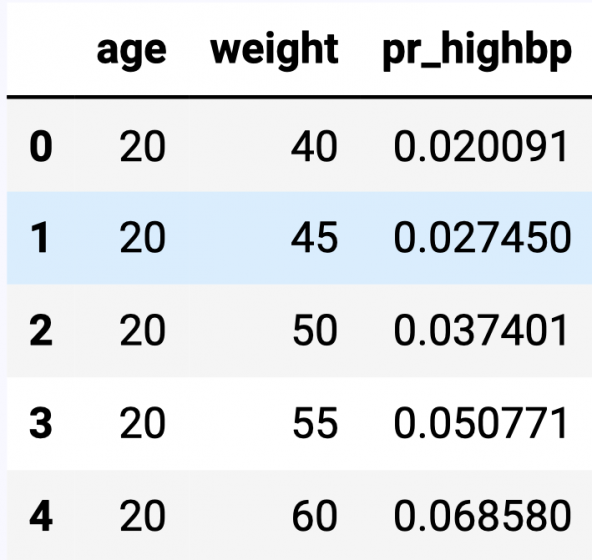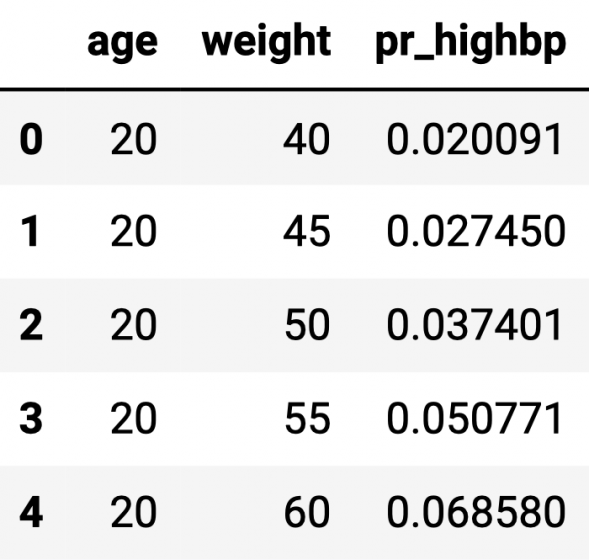## Jupyter Notebook with Stata

Jupyter Notebook is a powerful and easy-to-use web application that allows you to combine executable code, visualizations, mathematical equations and formulas, narrative text, and other rich media in a single document (a “notebook”) for interactive computing and developing. Jupyter notebooks have been widely used by researchers and scientists to share their ideas and results for collaboration and innovation.

Now, you can invoke Stata and Mata from Jupyter Notebooks with the IPython (interactive Python) kernel, meaning you can combine the capabilities of both Python and Stata in a single environment to make your work easily reproducible and shareable with others.

## Let’s see it work

In Jupyter Notebook, you can use two set of tools provided by the pystata Python package to interact with Stata:

1. Three IPython (interactive Python) magic commands: stata, mata, and pystata
2. A suite of API functions

Before showing you how to use these tools, we configure the pystata package. Suppose you have Stata installed in C:\Program Files\Stata17\ and you use the Stata/MP edition. Stata can be initialized as follows:

In :

import stata_setup

stata_setup.config(“C:/Program Files/Stata17”, “mp”)

```  ___  ____  ____  ____  ____ ©
/__    /   ____/   /   ____/      17.0
___/   /   /___/   /   /___/       MP—Parallel Edition

Statistics and Data Science       Copyright 1985-2021 StataCorp LLC
StataCorp
4905 Lakeway Drive
College Station, Texas 77845 USA
800-STATA-PC        https://www.stata.com
979-696-4600        stata@stata.com

Stata license: 10-user 4-core network perpetual
Serial number: 1
Licensed to: Stata Developer
StataCorp LLC

Notes:
1. Unicode is supported; see help unicode_advice.
2. More than 2 billion observations are allowed; see help obs_advice.
3. Maximum number of variables is set to 5,000; see help set_maxvar.

.
```

If you get output similar to what is shown above for your edition of Stata, it means that everything is configured properly; see Configuration for more ways to configure pystata.

## Call Stata using magic commands

The stata magic is used to execute Stata commands in an IPython environment. In a notebook cell, we put Stata commands underneath the %%stata cell magic to direct the cell to call Stata. The following commands load the auto dataset and summarize the mpg variable. The Stata output is displayed underneath the cell.

In :

%%stata

sysuse auto, clear

summarize mpg

```. sysuse auto, clear
(1978 automobile data)

. summarize mpg

Variable |        Obs        Mean    Std. dev.       Min        Max
-------------+---------------------------------------------------------
mpg |         74     21.2973    5.785503         12         41

.
```

Stata’s graphs can also be displayed in the IPython environment. Here we create a scatterplot of car mileage against price by using the %stata line magic.

In :

%stata scatter mpg priceNext, we load Python data into Stata, perform analyses in Stata, and then pass Stata returned results to Python for further analysis, using the Second National Health and Nutrition Examination Survey (NHANES II; McDowell et al. 1981).

NHANES II, a dataset concerning health and nutritional status of adults and children in the US, contains 10,351 observations and 58 variables and is stored in a CSV file called nhanes2.csv. Among hese variables is an indicator variable for hypertension (highbp) and the continuous variables age and weight.

We use pandas method read_csv() to read the data from the .csv file into a pandas dataframe named nhanes2.

In :

import pandas as pd

import io

import requests

data = requests.get(“https://www.stata.com/python/pystata/misc/nhanes2.csv”).content

nhanes2

Out :10351 rows ×58 columns

We load the dataframe into Stata by specifying the -d argument of the %%stata magic, and then within Stata, we fit a logistic regression model using age, weight, and their interaction as predictors of the probability of hypertension. We also push Stata’s estimation results displayed by ereturn list, including the coefficient vector e(b) and variance–covariance matrix e(V), into a Python dictionary called myeret by specifying the -eret argument.

In :

%%stata -d nhanes2 -eret myeret

logistic highbp c.age##c.weight

ereturn list

```. logistic highbp c.age##c.weight

Logistic regression                                    Number of obs =  10,351
LR chi2(3)    = 2381.23
Prob > chi2   =  0.0000
Log likelihood = -5860.1512                            Pseudo R2     =  0.1689

------------------------------------------------------------------------------
highbp | Odds ratio   Std. err.      z    P>|z|     [95% conf. interval]
-------------+----------------------------------------------------------------
age |   1.108531   .0080697    14.15   0.000     1.092827     1.12446
weight |   1.081505    .005516    15.36   0.000     1.070748    1.092371
|
c.age#|
c.weight |   .9992788   .0000977    -7.38   0.000     .9990873    .9994703
|
_cons |   .0002025   .0000787   -21.89   0.000     .0000946    .0004335
------------------------------------------------------------------------------
Note: _cons estimates baseline odds.

. ereturn list

scalars:
e(rank) =  4
e(N) =  10351
e(ic) =  4
e(k) =  4
e(k_eq) =  1
e(k_dv) =  1
e(converged) =  1
e(rc) =  0
e(ll) =  -5860.151218806021
e(k_eq_model) =  1
e(ll_0) =  -7050.765484416371
e(df_m) =  3
e(chi2) =  2381.2285312207
e(p) =  0
e(N_cdf) =  0
e(N_cds) =  0
e(r2_p) =  .1688631210670459

macros:
e(cmdline) : "logistic highbp c.age##c.weight"
e(cmd) : "logistic"
e(predict) : "logistic_p"
e(estat_cmd) : "logit_estat"
e(marginsok) : "default Pr"
e(marginsnotok) : "stdp DBeta DEviance DX2 DDeviance Hat Number Resi.."
e(title) : "Logistic regression"
e(chi2type) : "LR"
e(opt) : "moptimize"
e(vce) : "oim"
e(user) : "mopt__logit_d2()"
e(ml_method) : "d2"
e(technique) : "nr"
e(which) : "max"
e(depvar) : "highbp"
e(properties) : "b V"

matrices:
e(b) :  1 x 4
e(V) :  4 x 4
e(mns) :  1 x 4
e(rules) :  1 x 4
e(ilog) :  1 x 20
e(gradient) :  1 x 4

functions:
e(sample)

.
```

We can access e(b) and e(V) by typing myeret[‘e(b)’] and myeret[‘e(V)’], respectively, in Python. They are stored in NumPy arrays.

In :

myeret [‘e(b)’], myeret[‘e(V)’]

```(array([[ 1.03035513e-01,  7.83537342e-02, -7.21492384e-04,
-8.50485078e+00]]),
array([[ 5.29930771e-05,  3.50509317e-05, -6.97861002e-07,
-2.69423163e-03],
[ 3.50509317e-05,  2.60132664e-05, -4.74084051e-07,
-1.94299575e-03],
[-6.97861002e-07, -4.74084051e-07,  9.55811835e-09,
3.50377699e-05],
[-2.69423163e-03, -1.94299575e-03,  3.50377699e-05,
1.50887842e-01]]))
```

We use margins and marginsplot to graph predictions over age, which more clearly illustrates the relationship between age and the probability of hypertension.

In :

%%stata

quietly margins, at(age=(20(10)80))

marginsplot

```. quietly margins, at(age=(20(10)80))

. marginsplot

Variables that uniquely identify margins: age

.
```We use margins to estimate the predicted probability of hypertension for all combinations of age and weight for values of age ranging from 20 to 80 years in increments of 5 and for values of weight ranging from 40 to 180 kilograms in increments of 5. The option saving(predictions, replace) saves the predictions to a dataset named predictions.dta. Our goal is to use Python to create a three-dimensional surface plot of those predictions.

The dataset predictions.dta contains the variables _at1 and _at2, which correspond to the values of age and weight that we specified in the at() option. The dataset also contains the variable _margin, which is the marginal prediction of the probability of high blood pressure. We rename those variables to age, weight, and pr_highbp respectively.

Lastly we store the dataset in Stata as a pandas dataframe named preddata in Python by specifying the -doutd argument.

In :

%%stata -doutd preddata

quietly margins, at(age=(20(5)80) weight=(40(5)180))

saving(predictions, replace)

use predictions, clear

list _at1 _at2 _margin in 1/5

rename _at1 age

rename _at2 weight

rename _margin pr_highbp

```. quietly margins, at(age=(20(5)80) weight=(40(5)180)) ///
>     saving(predictions, replace)

.
. use predictions, clear
(Created by command margins; also see char list)

. list _at1 _at2 _margin in 1/5

+------------------------+
| _at1   _at2    _margin |
|------------------------|
1. |   20     40   .0200911 |
2. |   20     45   .0274497 |
3. |   20     50   .0374008 |
4. |   20     55   .0507709 |
5. |   20     60   .0685801 |
+------------------------+

. rename _at1 age

. rename _at2 weight

. rename _margin pr_highbp

.
```

We list the first five observations of the age, weight, pr_highbp columns within the dataframe.

In :

Out :Next we use Python to create a three-dimensional surface plot. First, we set the graph size to be 10 by 8 inches using the figure() method in the pyplotmodule of the Matplotlib package. We use the axes() method to define a three-dimensional set of axes named ax and draw the surfrace plot with the plot_trisurf() method. Next we set the ticks and labels for the x, y, and z axes. Last, we adjust the elevation and azimuth of the plot.

In :

import matplotlib.pyplot as plt

import numpy as np

# define the axes

fig = plt.figure(1, figsize=(10, 8))

ax = plt.axes(projection=‘3d’)

# plot

ax.plot_trisurf(preddata[‘age’], preddata[‘weight’], preddata[‘pr_highbp’],cmap=plt.cm.Spectral_r)

# set ticks and labels for x, y, and z axes

ax.set_xticks(np.arange(20, 90, step=10))

ax.set_yticks(np.arange(40, 200, step=40))

ax.set_zticks(np.arange( 0, 1.2, step=0.2))

ax.set_xlabel(Age (years)”)

ax.set_ylabel(Weight (kg)”)

ax.set_zlabel(Probability of Hypertension”)

# adjust the view angle

ax.view_init(elev=30, azim=240)

# show the plot

plt.show()We have demonstrated how to access Stata from Python, but you can also access Mata from Python using the mata magic; see The mata magic and Example 4: Work with Mata.

## Interact with Stata using API functions

We can also interact with Stata by using a suite of functions defined in the config and stata modules from the pystata Python package. We will demonstrate how to use these API functions to interact with Stata as an alternative to the %%stata magic command.

We import the stata module from pystata. Then call the pdataframe_to_data() method to load the pandas dataframe nhanes2 into Stata and call the run()method to fit the logistic regression model.

In :

from pystata import stata

stata.pdataframe_to_data(nhanes2, force=True)

stata.run(‘logistic highbp c.age##c.weight’)

```Logistic regression                                    Number of obs =  10,351
LR chi2(3)    = 2381.23
Prob > chi2   =  0.0000
Log likelihood = -5860.1512                            Pseudo R2     =  0.1689

------------------------------------------------------------------------------
highbp | Odds ratio   Std. err.      z    P>|z|     [95% conf. interval]
-------------+----------------------------------------------------------------
age |   1.108531   .0080697    14.15   0.000     1.092827     1.12446
weight |   1.081505    .005516    15.36   0.000     1.070748    1.092371
|
c.age#|
c.weight |   .9992788   .0000977    -7.38   0.000     .9990873    .9994703
|
_cons |   .0002025   .0000787   -21.89   0.000     .0000946    .0004335
------------------------------------------------------------------------------
Note: _cons estimates baseline odds.
```

Then we use the get_ereturn() method to store the e() results returned by the logistic command in Python as a dictionary named myeret2 and display e(b)and e(V) within it.

In :

myeret2 = stata.get_ereturn()

myeret2[‘e(b)’], myeret2[‘e(V)’]

Out :

```(array([[ 1.03035513e-01,  7.83537342e-02, -7.21492384e-04,
-8.50485078e+00]]),
array([[ 5.29930771e-05,  3.50509317e-05, -6.97861002e-07,
-2.69423163e-03],
[ 3.50509317e-05,  2.60132664e-05, -4.74084051e-07,
-1.94299575e-03],
[-6.97861002e-07, -4.74084051e-07,  9.55811835e-09,
3.50377699e-05],
[-2.69423163e-03, -1.94299575e-03,  3.50377699e-05,
1.50887842e-01]]))
```

Next we call the run() method to estimate the predicted probability of hypertension for all combinations of age and weight, save the prediction results in a dataset named predictions.data, and load the dataset into Stata and rename the variables.

In :

stata.run(”’

quietly margins, at(age=(20(5)80) weight=(40(5)180)) ///

saving(predictions, replace)

use predictions, clear

list _at1 _at2 _margin in 1/5

rename _at1 age

rename _at2 weight

rename _margin pr_highbp

”’)

```.
. quietly margins, at(age=(20(5)80) weight=(40(5)180)) ///
>     saving(predictions, replace)

.
. use predictions, clear
(Created by command margins; also see char list)

. list _at1 _at2 _margin in 1/5

+------------------------+
| _at1   _at2    _margin |
|------------------------|
1. |   20     40   .0200911 |
2. |   20     45   .0274497 |
3. |   20     50   .0374008 |
4. |   20     55   .0507709 |
5. |   20     60   .0685801 |
+------------------------+

. rename _at1 age

. rename _at2 weight

. rename _margin pr_highbp

.
```

We call the pdataframe_from_data() function in the stata module to store the age, weight, and pr_highbp in current dataset to a pandas dataframe named preddata2.

In :

preddata2 = stata.pdataframe_from_data(var=“age weight pr_highbp”)